Start typing, then use the up and down aroows to select an option from the list.

# Organic Chemistry

Learn the toughest concepts covered in Organic Chemistry with step-by-step video tutorials and practice problems by world-class tutors.

3. Acids and Bases

Now that we understand what an acid is, we need a method of quantifying which acids are stronger and which are weaker. pH doesn’t work for this, let me explain why:

## pH vs. pKa

1

### concept

Why we use pKa instead of pH.1m
Play a video:
0

The Ka (dissociation constant) describes the tendency of a molecule to break apart. In the case of acids, that specifically means donating protons, which is exactly what we are interested in knowing!

2

### concept

The relationship between equilibrium constant and pKa.2m
Play a video:
0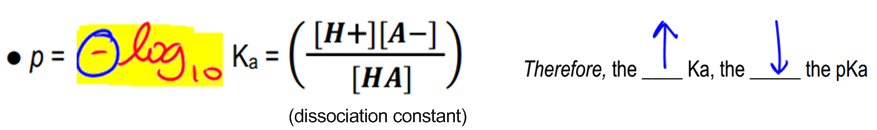3

### concept

The pH scale vs. the pKa scale.2m
Play a video:
0

The pH and pKa scales really are completely different. Pardon my French! pKas are obviously something I’m really passionate about.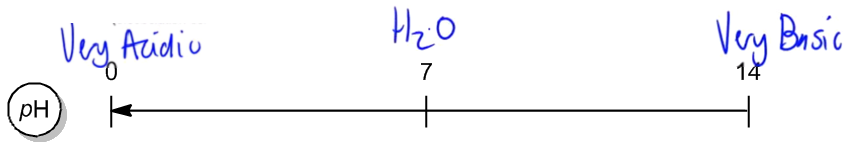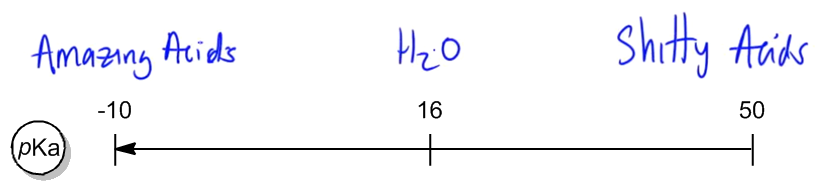## Calculating pKa

This is the easiest kind of question you could get. Calculating pKa’s just takes some very simple math.

What is the pKa of acetic acid? Hint:take the negative log of the dissociation constant.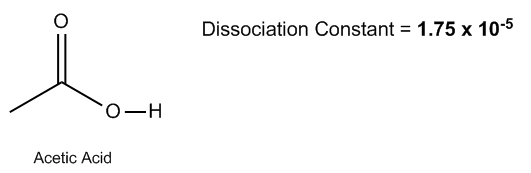4

### example

Calculate the pKa of acetic acid1m
Play a video:
0

What is the pKa of ammonium? Hint:take the negative log of the dissociation constant.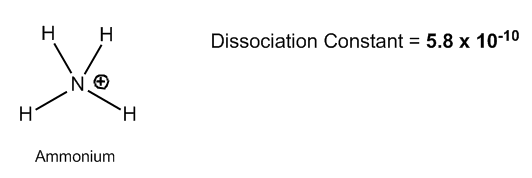5

### example

Calculating pKa and comparing acidity1m
Play a video: